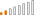cancel
Showing results for
Did you mean:Helper II

## Sum of distinct values, by category

Hello guys, I've been fighting a little bit with this new measure. This is my sample table:

 Category Quantity Cat 1 29 Cat 1 29 Cat 1 29 Cat 1 29 Cat 1 2 Cat 2 10 Cat 2 10 Cat 2 1 Cat 1 2 Cat 2 1 Cat 2 1 Cat 2 10 Cat 3 4 Cat 1 29 Cat 3 4 Cat 3 4 Cat 3 5 Cat 3 4

And all I want to do is to Sum The Quantity column but only the different numbers by Category. I mean, the output should be like this:

Cat 1 = 31

Cat 2 = 11

Cat 3 = 21

TOTAL = 63

Thank you guys, and have an excellent day!

1 ACCEPTED SOLUTIONContinued Contributor

hi,

you can try this measure:

`sum unique = SUMX(SUMMARIZE('Table', 'Table'[Category],'Table'[Required]), 'Table'[Required])`

below is the PBI file:

https://1drv.ms/u/s!Aps8poidQa5zk6sOB9ebWu-v8BHh5w

12 REPLIES 12Helper II
Measure = SUMX(DISTINCT(Table2[Quantity]),Table2[Quantity])Helper II

Hi @ .. I know you know a lot of DAX.. and you've helped me before... Do you know how to do this??

Sorry for bothering you!.. And thanks a lot!Anonymous
Not applicable

This should do it:

```Sum Distinct =
CALCULATE(
SUMX(
SUMMARIZE('Sample data', 'Sample data'[Quantity]),
'Sample data'[Quantity]
)
)```

The "summarize" will give you a table with only the distinct values, which are then added by the SUMX.

Hope this helps!

ScottResident Rockstar

@Anonymous

Be sure to group by Category, not Quantity in the summarize. You can also get rid of the CALCULATE part of the expression, since you don't want to change filter context.Anonymous
Not applicable

Hi Cmcmahan,

didn't want to put "category" in the calculation, because that hard codes it to work only for categories. The way I've writen it, it will give you the sum of distinct values for anything you put on the visualization - categories, departments, months, whatever you want.

You are correct I could probably get away without the CALCULATE - it's habit.

Thanks,

ScottAnonymous
Not applicable
```Column_SUM =
CALCULATE ( SUM( T15[Quntity] ), ALLEXCEPT ( T15, T15[Category] ) )```

Enjoy!
AHelper II

@AnonymousHi.. any thoughs?

ThanksResident Rockstar

What sort of visual are you putting this in?

It looks like a basic table, but if you were using just the category field and a measure, you wouldn't have repeats.  Are you also showing other data in the table like an ID/index or something?

Could you take a screenshot of the current table you have and the fields in the values bucket of the visualization pane?Helper II

In fact, it is more complacated than it looks, you are right, we should be able to use the measure with other kind of columns, like dates, categories, subcategories, etc.. I'll try to explain the whole issue..

This is my table: (i'm just putting some of the table fields). It is about candidates I need to hire for my companyFirst, The "Required" field refers to the number of candidates I need for a specific subcategory. If I want to know how many I've hired, I do this:

Hired Candidates =
CALCULATE(
COUNT(
Table[Required]); Table[Process State] = "19. PERSONAL HABILITADO")

Process State refers to the State that the candidate is in the process.. State "19. PERSONAL HABILITADO" is when the candidate is finally hired..

Then, I want to know how many candidates I need for every subcategory, or category, or priority, etc.. but the thing is, as you can see, that, for every row.. it shows the same number of candidates needed for every subcategory.. obvious, The measure I need, it is necessary to be just for the different values.. I mean..

Now, lets say I need 24 candidates for Category "AYUDANTE DE CAMPO" so I put "24" in Required field.. but then, we require 3 more candidates, so, in the next row it will be necessary to put "3" in the "required" field . so, finally, I need 27 candidates for that specific Category..

What I need is a measure that SUM the "Required" field, but just the values that are not repeated..

EX:

Category   Required

Cat 1              2

Cat 2              2

Cat 2              2

Cat 2              4

Cat 1              5

The final result would be:

CANDIDATES REQUIRED = 13

REQUIRED IN Cat 1: 7

REQUIRED IN Cat 2: 6

I know the table is not as efficient as it should be.. but thats what I have by now..

The reason why I want to know that information (the measure) is because, after that, I will calculate how many candidates do i need to fulfill the requirement..

Candidates needed = Candidates required - candidates hired

The VISUAL I will use is a "Matrix table"... In column I will use "Date" ... that refers the day the candidates should start working..

I hope I explain myself.. THANK YOU GUYS!

Omar.Continued Contributor

hi,

you can try this measure:

`sum unique = SUMX(SUMMARIZE('Table', 'Table'[Category],'Table'[Required]), 'Table'[Required])`

below is the PBI file:

https://1drv.ms/u/s!Aps8poidQa5zk6sOB9ebWu-v8BHh5wResident Rockstar

If you're trying to set this up as some sort of calculated table, I'd use SUMMARIZE.

`SummaryTable = SUMMARIZE(T15, T15[Category], "Qty", SUM(T15[Quantity))`Helper II

@AnonymousThank you! but I just used it and it doesn't work!

That measure took all the values .. I need it to take single values for every category.. I mean:

category     quantity

Cat1                10

Cat1                10

Cat1                10

Cat1                 2

Cat2                 4

Cat2                 4

Cat2                 2

Cat3                 1

Cat3                 1

Cat3                 1

Cat3                 4

The result should be like this:

Cat1: 10 + 2 = 12( I need it to take just the values 1 time, not repeated)

Cat2: 4 + 2 = 6

Cat3: 4 + 1 = 5

Total = 12 + 6 + 5 = 23

The measure should allow me to use it as in TABLE but also as a CARD visual..